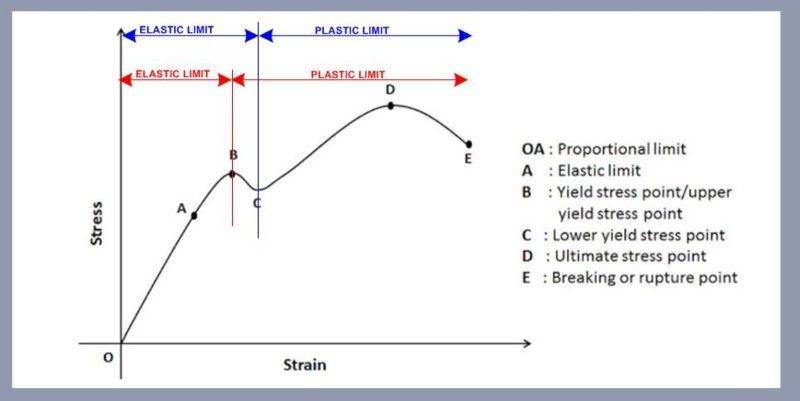# Understanding Yield Strength & Stress Strain Curve

• tomtomtom1
In summary, the conversation discusses the definitions of yield strength and yield point, as well as the difference between the two points shown on a stress-strain curve. The speaker also mentions the work hardening of materials in the plastic deformation zone and provides a graph for better understanding. The limits of elastic and plastic deformation are said to commence from the limits in red on the graph. The speaker cannot explain why the limits in blue are shown on the graph.

#### tomtomtom1

Hi all

I was hoping someone could shed some light on the following:-

I am trying to understand what Yield strength is and understand the exact limit of where elastic and plastic deformation occurs on a stress strain curve.

Correct me if I am wrong but I define:-

Yield strength as the amount of stress a material can undergo before it deforms plastically.

Yield Point
is the point on the stress strain graph where Elastic deformation ends and Plastic deformation begins.

Would you agree with the above definitions, the reason why I ask is because on a stress strain curve (as shown below), we have 2 x Yield Stress Points shown as B and C.

1) what is the difference between these points.
2) where would the elastic and plastic limits commence from, the limits in Red or the limits in Blue?#### Attachments

I think the portion of the curve after point B is caused by work hardening of the material during elongation.Where:
Pass point 2 (B on your graph), you are in the plastic deformation zone (thus, the limits in red on your graph would make sense). It is interesting to see the same stress-strain curve based on the actual area ##A## instead of the initial area ##A_0## (blue line B). Even though the relationship is not as linear in the plastic zone, it still requires an increase of the actual stress to get an increase in strain.

I can't explain why they wrote the limits in blue on your graph.

•Chestermiller

## What is yield strength?

Yield strength is the maximum amount of stress that a material can withstand before it starts to deform permanently. It is often used as an indicator of the strength and durability of a material.

## How is yield strength measured?

Yield strength is typically measured by conducting a tensile test on a sample of the material. This involves applying a gradually increasing amount of stress to the material until it starts to deform. The yield strength is then determined by identifying the point where the material begins to deform permanently.

## What is the stress-strain curve?

The stress-strain curve is a graphical representation of the relationship between stress and strain in a material. It shows how a material responds to an applied force, and can provide valuable information about its strength and stiffness.

## What does the stress-strain curve tell us about a material?

The stress-strain curve can tell us about a material's stiffness, strength, ductility, and resilience. By examining the shape of the curve, we can determine the yield strength, ultimate tensile strength, and other important properties of the material.

## How is the stress-strain curve used in engineering?

The stress-strain curve is an essential tool in engineering as it helps us understand how materials behave under different levels of stress. It is used to select the appropriate material for a given application, design structures that can withstand specific loads, and predict the failure of materials under certain conditions.